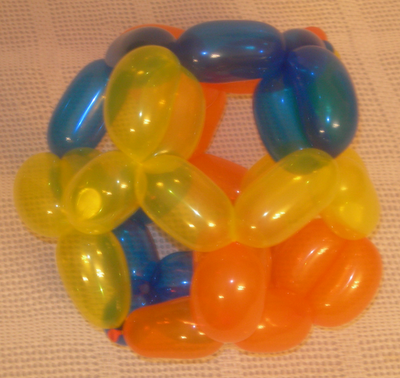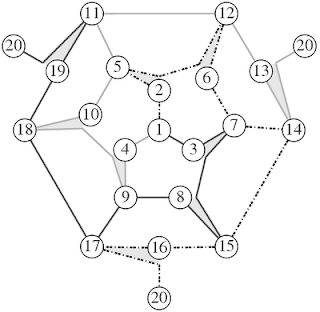## Sunday, August 24, 2008

### The Balloon DodecahedronThe dodecahedron is a tricky structure to make out of a small number of balloons. There are twenty degree 3 vertices and thirty edges. For symmetry, we need the number of balloons to divide the number of edges but as well figure out how to deal with the odd degree vertices. I have designed a 2-balloon dodecahedron but it was not very aesthetically pleasing. The design I currently prefer is a 3-balloon design. The trick was to instead of using all the ends of the balloons to each take care of an odd degree vertex leaving fourteen (which is not divisible by three), was to have two vertices where three ends come together leaving eighteen vertices of degree 3 (which is divisible by three).

In this design, I use nine doubled edges. The doubled edges stabilize the shape as well as locking the balloons local geometry. To make the weaving of the balloons easier, the doubling of the edges are delayed until the other balloons are in place. This delay reduces the tension on the twisting.

Below is a video of the construction of a dodecahedron.

Here is the psuedo-gauss code for this design.

1a 2 5 2d 6 12 6e 7b 14 15e 16 17 16 20
1a 3 7b 3 8 15 8e 9c 17 18e 19 11 19 20
1a 4 9c 10 18e 10 5d 11 12e 13 14 13 20

* the superscript means a delayed connection. For example in the first balloon, the first line, the sequence 2 5 2d 6 12 6e 7b can be created all at once as a string of 7 segments like a string of sausages. Even though the 2 is repeated, it is not joined until the third balloon reaches the d (see the 5d). Then the two segment 2-5 and 5-2d can be twisted together to form a double edge.The graph has a three-fold rotational symmetry (C3). To keep the symmetry of the graph, node 20 has three copies but really it is a single node at infinity.